08/03 11:44

# 一、数据集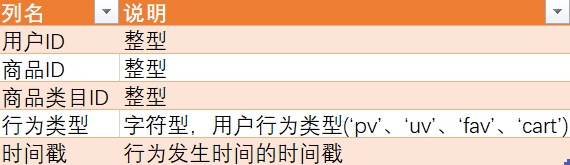# 二、提出问题

## 1.用户购物情况

PV(总访问量),UV(用户总数)，日均访问量，跳失率，用户各种行为类型，复购率分别是多少。

# 三、数据清洗

``````#导入相关包
import pandas as pd
import numpy as np
import time
#导入数据
#设置列名
data_origin.columns = ['User_Id','Item_Id','Category_Id','Behavior_type','Timestamp']
#观察数据
#观察缺失值
#data_origin.isnull().sum()
index_lose = data_origin[data_origin['Timestamp'].isnull()].index
data_origin.iloc[index_lose,:]
#可以看到，缺失时间戳的那一行Behavior_type也是异常，因此决定丢掉这一行
data = data_origin.dropna(axis = 0)
#此时Timestamp列为科学计数法显示，将其改成整型
data[:,'Timestamp']=data['Timestamp'].astype(int)
data.reset_index(drop=True,inplace=True)
#时间戳列转换为日期、时间数据。并把日期和时间分为两列
data.loc[:,'Timestamp']=data['Timestamp'].apply(lambda x:time.strftime('%Y-%m-%d %H:%M:%S',time.localtime(x)))
data.loc[:,'Date']=data['Timestamp'].apply(lambda x:x.split(' '))
data.loc[:,'Time']=data['Timestamp'].apply(lambda x:x.split(' '))
#删除时间戳列
data=data.drop(columns='Timestamp',axis=1)
#我们只取日期为2017-11-25到2017-12-03之间的数据，查看一下这个时间段之外的具体情况
data[data['Date']<'2017-11-25'].shape
data[data['Date']<'2017-11-25']['Date'].value_counts()
data[data['Date']>'2017-12-03'].shape
data[data['Date']>'2017-12-03']
#时间区间外的日期数据剔除
data=data[(data['Date']>='2017-11-25')&(data['Date']<='2017-12-03')]
#查看重复值
data[data.duplicated()]
#删除重复值
data.drop_duplicates()
#重置索引
data.reset_index(drop=True,inplace=True)
#数据清洗完成，导出数据
data.to_csv(r'F:\taobao\UserBehavior_Done.csv',index=False)``````

# 四、导入SQL

``````#建立数据库
create database taobao;
#建立表格
user taobao;
#创建表格
create table user (
user_id int not null,
item_id int not null,
category_id int not null,
behavetype varchar(10) not null,
dates date not null,
times time not null);
#导入数据
INTO TABLE USER
FIELDS TERMINATED BY ','
IGNORE 1 LINES;``````

# 1、用户流量参数及漏斗模型

## PV（总访问量）

``````SELECT COUNT(behavetype) AS 每日访问量,dates
FROM USER
WHERE behavetype = 'pv'
GROUP BY dates
ORDER BY dates``````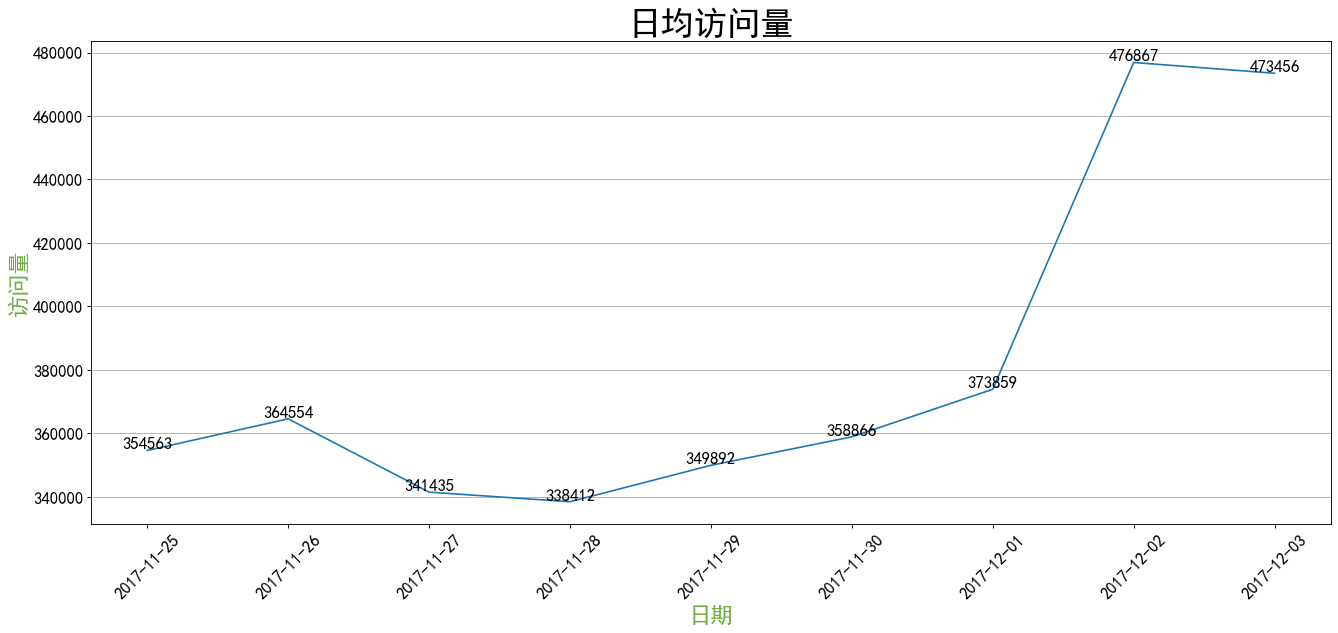## UV（访问用户总数）

``````SELECT COUNT(DISTINCT user_id) AS 总人数
FROM USER
#总用户数 37376``````

## 跳失率

``````SELECT COUNT(user_id) AS 只有点击行为的用户 FROM(
SELECT user_id
FROM USER
GROUP BY user_id
HAVING COUNT(DISTINCT behavetype) = 1) a;``````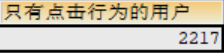## 用户行为视观表

``````#观察用户行为
CREATE VIEW user_acts AS
SELECT user_id,COUNT(behavetype) AS 行为次数,
SUM(CASE WHEN behavetype = 'pv' THEN 1 ELSE 0 END) AS 点击次数,
SUM(CASE WHEN behavetype = 'fav' THEN 1 ELSE 0 END) AS 收藏次数,
SUM(CASE WHEN behavetype = 'cart' THEN 1 ELSE 0 END) AS 加购次数,
SUM(CASE WHEN behavetype = 'buy' THEN 1 ELSE 0 END) AS 购买次数
FROM USER
GROUP BY user_id
ORDER BY 行为次数 DESC;
#查看用户购物情况
SELECT * FROM user_acts;``````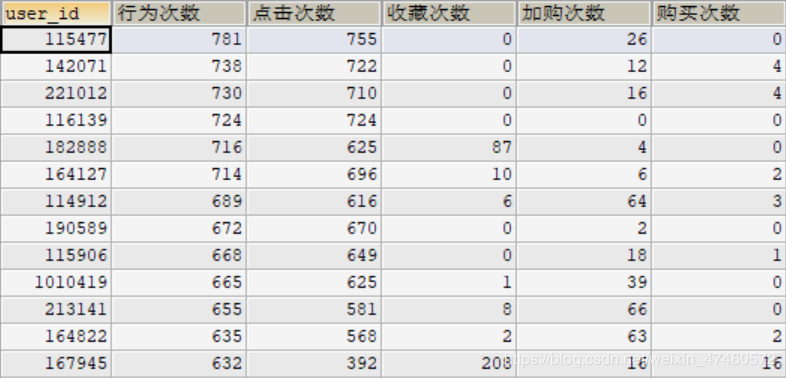## 漏斗模型

``````SELECT
CONCAT(ROUND(SUM(点击次数)/SUM(点击次数)*100,2),'%') AS pv,
CONCAT(ROUND((SUM(收藏次数)+SUM(加购次数))/SUM(点击次数)*100,2),'%') AS pv_favCart,
CONCAT(ROUND(SUM(购买次数)/(SUM(收藏次数)+SUM(加购次数))*100,2),'%') AS favCart_pv
FROM user_acts;``````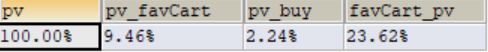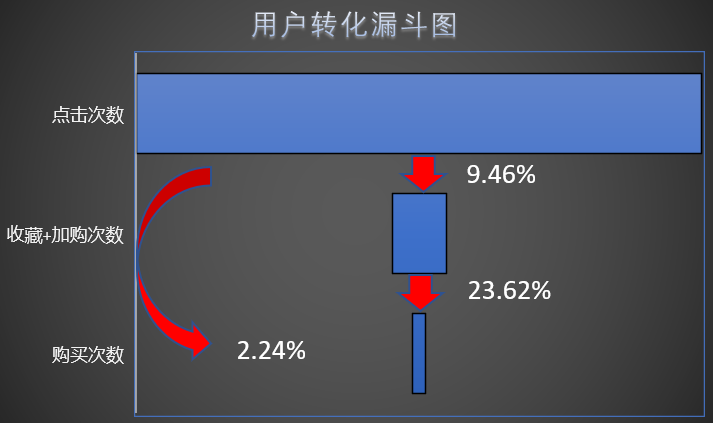## （1）浏览及收藏次数最多的用户

``````SELECT user_id,收藏次数+加购次数 AS 收藏及加购次数
FROM user_acts
GROUP BY user_id
ORDER BY 收藏及加购次数 DESC;``````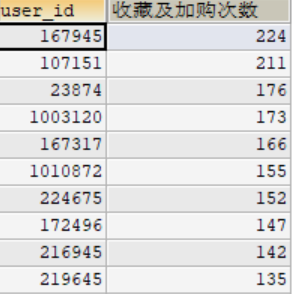此类用户可能购买能力不够强，可以针对对此类用户多投放优惠券，刺激他们的购买欲望。

## （2）购买次数最多的用户

``````SELECT user_id,购买次数
FROM user_acts
GROUP BY user_id
ORDER BY 购买次数 DESC;``````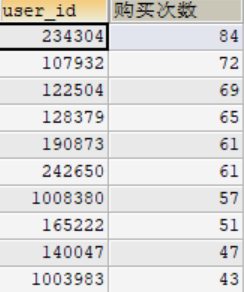## （3）用户复购率

``````SELECT
SUM(CASE WHEN 购买次数>1 THEN 1 ELSE 0 END) AS 复购的用户,
SUM(CASE WHEN 购买次数>0 THEN 1 ELSE 0 END) AS 购买的用户总数,
CONCAT(ROUND(SUM(CASE WHEN 购买次数 > 1 THEN 1 ELSE 0 END)/SUM(CASE WHEN 购买次数 > 0 THEN 1 ELSE 0 END)*100,2),'%') AS 复购率
FROM user_acts``````

# 3、不同购买率用户的特征

## （1）高购买率人群特征

``````SELECT user_id,点击次数,收藏次数,加购次数,购买次数,
SUM(购买次数)/SUM(点击次数) AS 购买率
FROM user_acts
WHERE 点击次数 > 0
GROUP BY user_id
ORDER BY 购买次数 DESC``````

## （2）购买率低的人群特征

``````SELECT user_id,点击次数,收藏次数,加购次数,购买次数,
SUM(购买次数)/SUM(点击次数) AS 购买率
FROM user_acts
WHERE 点击次数 > 0
GROUP BY user_id
ORDER BY 购买次数 ASC``````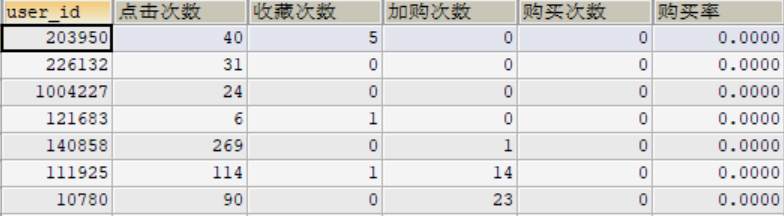# 4、不同时间用户的活跃度

## （1）一天用户活跃度

``````#给原表user加入新列hours
ALTER TABLE USER ADD hours CHAR(10) NULL;
UPDATE USER
SET hours =LEFT(times,2);
#用户活跃时间段视图
SELECT hours,COUNT(behavetype) AS 用户行为总量,
SUM(CASE WHEN behavetype = 'pv' THEN 1 ELSE 0 END) AS 点击次数,
SUM(CASE WHEN behavetype = 'fav' THEN 1 ELSE 0 END) AS 收藏次数,
SUM(CASE WHEN behavetype = 'cart' THEN 1 ELSE 0 END) AS 加购次数,
SUM(CASE WHEN behavetype = 'buy' THEN 1 ELSE 0 END) AS 购买次数
FROM USER
GROUP BY hours
ORDER BY hours;``````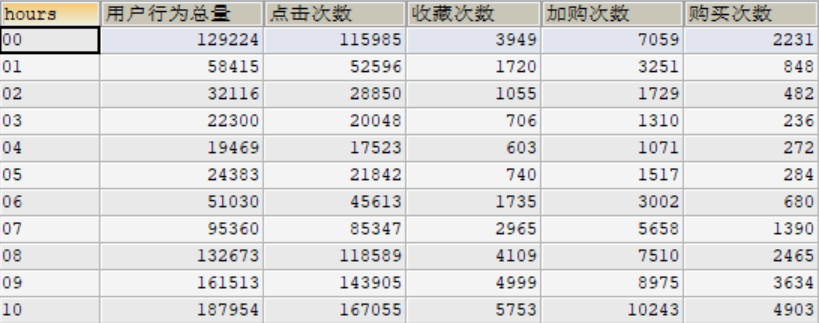## （2）一周用户活跃度

``````CREATE VIEW weeks AS
SELECT DATE_FORMAT(dates,'%W')AS 星期,COUNT(behavetype) AS 用户行为总量,
SUM(CASE WHEN behavetype = 'pv' THEN 1 ELSE 0 END) AS 点击次数,
SUM(CASE WHEN behavetype = 'fav' THEN 1 ELSE 0 END) AS 收藏次数,
SUM(CASE WHEN behavetype = 'cart' THEN 1 ELSE 0 END) AS 加购次数,
SUM(CASE WHEN behavetype = 'buy' THEN 1 ELSE 0 END) AS 购买次数
FROM USER
WHERE dates BETWEEN '2017-11-25' AND '2017-12-01'
GROUP BY 星期
ORDER BY 星期

SELECT * FROM weeks
ORDER BY 星期 ``````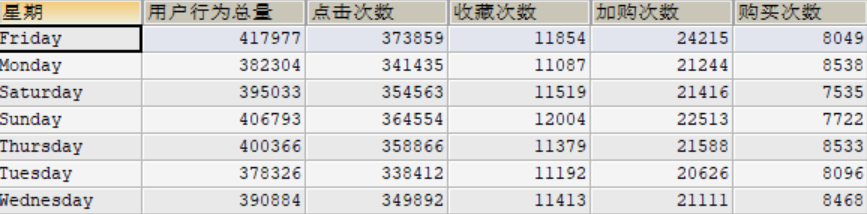0
0 收藏

### 作者的其它热门文章0 评论
0 收藏
0